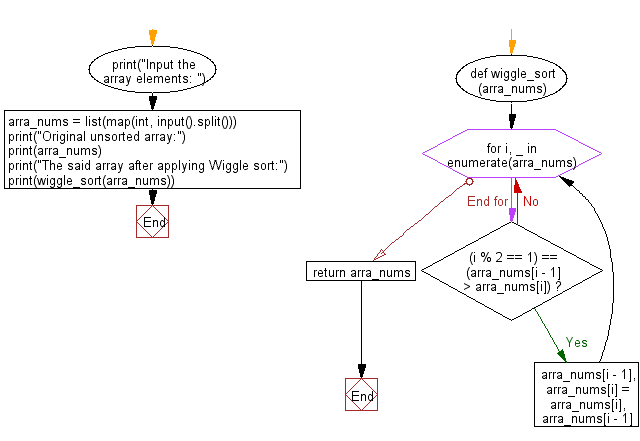﻿ Python: Sort an unsorted array numbers using Wiggle sort - w3resource# Python: Sort an unsorted array numbers using Wiggle sort

## Python Search and Sorting: Exercise-24 with Solution

Write a Python program to sort an unsorted array numbers using Wiggle sort.

Wiggle Sort:
Given an unsorted array nums, reorder it in-place such that nums <= nums >= nums <= nums....
For example, given nums = [3, 5, 2, 1, 6, 4], one possible answer is [1, 6, 2, 5, 3, 4].

Sample Solution:

Python Code:

``````def wiggle_sort(arra_nums):
for i, _ in enumerate(arra_nums):
if (i % 2 == 1) == (arra_nums[i - 1] > arra_nums[i]):
arra_nums[i - 1], arra_nums[i] = arra_nums[i], arra_nums[i - 1]

return arra_nums

print("Input the array elements: ")
arra_nums = list(map(int, input().split()))
print("Original unsorted array:")
print(arra_nums)
print("The said array after applying Wiggle sort:")
print(wiggle_sort(arra_nums))
```
```

Sample Output:

```Input the array elements:
1 5 2 3 4 6
Original unsorted array:
[1, 5, 2, 3, 4, 6]
The said array after applying Wiggle sort:
[1, 5, 2, 4, 3, 6]
```

Flowchart:Python Code Editor:

What is the difficulty level of this exercise?

Test your Programming skills with w3resource's quiz.

﻿

## Python: Tips of the Day

The Zip() Function:

```>>> students = ('John', 'Mary', 'Mike')
>>> ages = (15, 17, 16)
>>> scores = (90, 88, 82, 17, 14)
>>> for student, age, score in zip(students, ages, scores):
...     print(f'{student}, age: {age}, score: {score}')
...
John, age: 15, score: 90
Mary, age: 17, score: 88
Mike, age: 16, score: 82
>>> zipped = zip(students, ages, scores)
>>> a, b, c = zip(*zipped)
>>> print(b)
(15, 17, 16)
```